## Wednesday, 22 June 2011

### Notes uploaded after the notes season summer/2011

Economics
Money supply
Money model

Chemistry
Integrated the whole part of carbon chemistry:
-nomenclature and physical properties
-isomerism
-reactions
-applications
Enthalpy

Physics
Electrostatics
Current electricity
Electromagnetism I
Elective: Astronomy
-Planetary motion (mechanics)
-Stars and universe

Mathamatics
Combinatorics

## Tuesday, 21 June 2011

### Physics notes: Electromagnetism I

Electromagnetism
-          Unlike pole attract each other while like poles repel each other.
-          They exist in pairs, the magnets contain N and S pole at the same time while magnetic monopole does not exist in classical physics.
-          The magnetic field points from N to S, it's the direction of force acting on a hypothesized N monopole.
-          Magnetic field density B, often referred as magnetic field, has unit Tesla (T). It's proportional to the field lines on the diagram.

They are measured by two instruments:
1) Hall probe for fixed B; 2) search coil for varying B over time
3) Test current balance and 4) Other coil in various set up
When current passes through a conductor, magnetic field is induced following the right hand grip rule. A current out of paper would have anti-clockwise magnetic field, and opposite current gives opposite B direction. The magnetic field due to current in conductor is given by B = μ0I/2πr, where I is the current, r is the distance from the conductor, μ0 = 4π*10-7 Tm/A. is the magnetic constant B is a vector and can be added through vector sum.

When we bend the conductor into a series of circle, which is called solenoid, we have a uniform magnetic field inside the solenoid. B inside the solenoid is given by B = μ0nI = μ0IN/L. Note that n=N/L, N is the turns of solenoid and L is its length. It's noted that radius of solenoid r<<L.
At two ends of the solenoid, B is just half as that of inside the solenoid, and out of the solenoid, B decreases gradually to zero due to flux leakage.
When a segment of conducting wire is placed in the uniform magnetic field, the force experienced is given by F = L(B x I) (the cross product) = BILsinθ. The direction of F can be given by the Fleming's left hand rule.

Consider the force per unit length between two conducting wire, assume they are parallel:
F/ L = BI'L = (μ0I/2πr )I' = μ0II'/2πr, it follows that when the direction of current are the same, the force is attractive.
1 A can be defined as the current which flowing in each of the two infinitely long parallel straight conductors of negligibly small cross-sectional area, separated 1 m in vacuum, exerting 2*10-7 Nm-1 on each other.
In a current balance, there's a rectangular conducting loop, but one side is insulated in order to balance the weight of the loop. Place a pair of slab magnet on the conducting side so that there's upward force acting on the conductor. By putting extra rider on the loop we are able to calculate the magnitude of magnetic force, then we can calculate the B field strength.

Motor
1)       The original motor
As in the figure, it rotates due to the moment acting on the coil. However it tends to stop at perpendicular state since the force is balanced and when the coil turns, the force acting on the coil tends to push back the coil to the perpendicular state.

2)       Improved motor
A commutator is applied so that the direction of current in the coil is inversed when the coil passes through the perpendicular axis of coil. Then the coil spins simultaneously. The disadvantage is that the angular acceleration is unstable, and then its speed is unstable.

Consider the couple along the spinning axis,
τ = 2Fs = 2(BIL)(w/2 sinθ) = NBAI sinθ, where w is the width of coil, A is the area of coil, θ is the angle between coil and vertical plane. It follows that F = 0 when it's perpendicular. It spins due to inertia.
3)       Practical motor
We use concave cylindrical magnet such that a radial magnetic field is set up. Then B perpendicular to I, τ = NBAI, then its acceleration is stable.
In practical, there're three pairs of coil in the ammature and soft iron core inside it.
We can increase the magnitude of force by:
-          Increase number of loops of coil, intensify B field or current passing through.
-          Lengthen the magnet (if it's shorter than the coil), add soft iron core or lengthen the width.
These method can be proved by τ = NBAI or the concept of magnetic flux.
Motion of charged particles in a magnetic field
Charge carrier is a microscopic view on conductors:
-          Electrolyte: positive and negative ions
-          Vacuum tube: electron, discharge tube: electron and positive ions
-          Metal: free electron (delocalized)
-          N-type semiconductor: positive holes; P-type semiconductors: free electrons
Definition of current: I = dQ/dt = neva (A generalized form nqva, where n is the number charge carrier per unit volume, q is the charge per unit carrier, v is the drift velocity, a is the cross-sectional area of conductors. When the charge carrier is electron we write as neva for simplicity.) It refers to charge passing through a point in a unit of time.
Drift velocity is the net movement of electron. Due to thermal motion it has the speed of 106ms-1, but the net movement is zero. Under p.d. or electric field, it has a net movement of about 10-4ms-1, which causes the current to occur. At the same time, the drift velocity along the wire is unique since once the circuit is open, electrical signal transmits about the speed of light so that all charge carriers stop drifting at the same time.
When a magnetic force is produced on a conducting wire, microscopically we say magnetic force is acting on the charge carriers. Assume N is the number of charge carriers in a piece of conductor, by n = N/AL, I = nqvA = (N/AL)qvA = Nqv/L, force acting on the conductor = force acting on all the charge carriers = BILsinθ = B(Nqv/L)Lsinθ = N(qvBsinθ)
Therefore the magnetic force acting on a single charge carrier F = qvBsinθ, or F = q(v x B).

1)       When v is perpendicular to B
In this case, F = qvB which is perpendicular to v and B, then the charge performs a circular motion until it goes out of the magnetic field in opposite direction and same speed.
2)       When v is parallel to B
In this case, F = 0, the charge carrier is not affected by the B field, so it undergoes rectilinear motion.
3)       Other case
We put the velocity vector into two components: perpendicular and parallel to the B field. The perpendicular sector is affected by B field so that it undergoes circular motion, at the same time the parallel component is unaffected, combining gives a helical motion.

Hall voltage: the phenomenon of generating voltage under magnetic field in direction perpendicular to the flow of current.
Consider the following figure. Due to magnetic force, the charge carriers are deflected and accumulate in the two sides of the conductor so that one side is relatively positively charged and another side is relatively negatively charged. Then a electric field is set up between the two plates and the electric force acting on the charge carriers is opposite to the magnetic force. When FE=FM, a steady p.d. is set up between the two plates.
Note that the polarity of two plates are the same no matter the charge carrier is positive or negative since when the polarity of charge reversed, force acting on it and the deflecting direction is reversed. Positive charge going to the left is same as negative charge going to the right.
Balancing the force: qE = qV/d = qvB, E = VH/d = vB = (I/nqA)B = IB/nqdt, we have VH = IB/nqt, where VH is the hall voltage and t is the thickness of conductor (the dimension that parallels B)

It can be used to measure the magnitude of magnetic field. In order to raise the sensitivity, semiconductor is used so that n is much smaller, while VH is still measured in mV. The apparatus is called Hall Probe, where a semiconductor is connected with a d.c. supply, and vertically connected with a millivoltmeter, then voltage is registered when B field is passing through (perpendicular to I and B). Besides, the connection of millivoltmeter may not be accurate so potentiometer is used to offset the unexpected p.d.. It's connected across the semiconductor.
Precautions when measuring B-field
-          Make sure the orientation is correct. For example, in hall probe, adjust its direction so that B field to be measured is perpendicular to I and B.
-          Measure in the east-west orientation if possible so that the B field due to the Earth (N-S) does not affect your result.
Some applications on electromagnetism: maglev train, speakerphone, mass spectrometer, speed selector.

## Saturday, 18 June 2011

### Area maximization problem and its variations

First of all, I would like to point out that this is my first time using LaTeX in my blog by iserting image. i don't know why I can't insert LaTeX image by using the official js document. So if you have any ideas plz tell me.

This is a typical question in differentiation topic.
A rectangle is inscribed in an isosceles triangle with base 12 cm and height 10 cm. Find the maximum possible area of the rectangle.

Method 1: Differentiation
This is the most proper way to solve it in secondary level.
Let the height be x cm, then the width is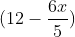, Considering area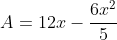, set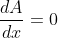, we can get x = 5.
Then A = 30, the maximized area is.

You may notice that the height is as half of the original triangle, so you may wonder are there any other method to deal with this problem...

Method 2: The logic of similar figures
Lemma: When we inscribed similar figures in another consecutively, the ratio of area between the two similar figures are equal.
For example, we have a circle of radius x, now inscribe a square inside, inscribe another circle inside the square and a square in the smaller circle.
Area of Circle 1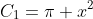Area of Square 1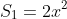Area of Circle 2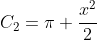Area of Square 2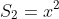Obviously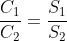The proof is quite simple: they both involve two steps "inscribe square in circle" and "inscribe circle in square". Putting these two steps in different order gives the above result.
Now, you may try to inscribe a triangle in the rectangle, that obviously gives the area ratio of 1/2.
Next, you focus on the area ratio between the large and smaller triangles. The two conditions:
1) Similar figures
2) smaller triangle can be inscribed in the rectangle.
Then you can show that the inscribed triangle is similar to the large one if and only if it's 1/4 of original. Otherwise the dimension of rectangle won't fit the triangle with similar shape.
Therefore, Area ratio of triangle : area of rectangle = 1/2 : 1/4, so area of rectangle = 12*10/4 =Method 3: Area inequlities
Consider the area of triangles (out of the rectangle).
Let the base be k cm, the base of rectangle be (k-x) cm. Then we can show that the sum of areas of triangle is given by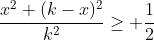Since the inequlities is equivalent to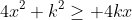which is true by AM-GM inequilities.
Equility holds when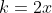, x = 6, then the base of rectangle is half of the triangle, which gives similar result of above.

Method 4: The concept of elasticity
If you study economics, you must heard of the concept of elasticity, and one of its function is to measure the total revenue obtained (PQ) along the demand curve.
Elasticity = % change in P / % change in Q.
Now we are going to give the mathematical frame of this tool.
P is on the y-axis, Q is on the x-axis, % change refers to dx/x
Then we define the elasticity of a curve on the cartesian plane given by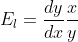.
Focus on the first quadrant. Consider the following fact:
1) Economical fact: Profit will not maximized infinitely by increasing/decreasing the price.
Mathematical fact: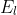changes along a stright line since x,y value varies.
2) Economial fact: Elasticity determine the gain and lose of TR. When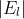<1, a raise in price rise TR. When>1, a rise in price decreases TR. In= 1, the change in price does not change TR.
Mathematical proof: We sayis the ratio of gain and lose in area enclosed due to a infinitely small change in y value. If needs more technique of partial differentiation so we won't discuss that here.
3) Economical fact: TR maximizes as the equilibrium stays at mid-point of the demand curve.
Mathematical proof: For a stright line, we are able to show that the part below and above the mid-point is elastic and inelastic respectively. Then staying in the mid-point maixmizes the area enclosed.
4) Economical fact: the elasticity of curve at mid-point is 1.
Mathematical proof: Consider a line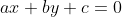. (a,b>0, c<0 so that it passes the first quadrant), then the mid-point between the intercepts is given by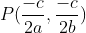while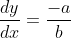, putting them intogives 1.

Combining the above result, we can show that when the height of rectangle is half of that triangle, the area is maximized.

To be honest we don't have much application related to elasticity, but it can be a kind of practices in differential equations.
e.g. Find the integral curve if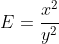Solution: putting this into the definition of elasticity, we have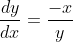,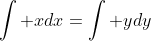,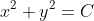, where C is a constant.

Ex.
1) Prove that a curve with elasticity 1 is hyperbolic considering the hyperbolic curve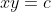.
2) Show that the elasticity of curve is independent of a linear transformation.
3) Describe the elasticity of a ellipse.
4) Describe the elasitcity of a logarithmetic function.
5) Find the curve if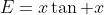6) Find the curve if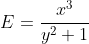7) Find the curve if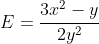(difficult!)
8) Find the maximum possible area of a triangle inscribed in a equilangular hexagon of side length 1,2,3,4,5,6 (the order of side iwth different lengths can be permuted as long as an enclosed hexagon is formed)

9) Prove that there does not exist polyhedron with equal side length and equal face area.
10) Prove or disprove the existance of polyhedron that when turncated (as you like), it has equal side length and euqal face area (excluding the face produced during turncation).

## Saturday, 11 June 2011

### TWC Final TW 5:4 JP

Yeah late video. As the referee of the final match that's really exciting and I was just to press the keys to dance with the beautiful melody... lol

## Thursday, 9 June 2011

### Economics: Money Market

Money market
Different people may have different combinations of assets (portfolio) like money, flats, shares, etc. We analysis the relationship between the nominal interest rates and the real output of goods and services in the market by this model.
People keep their assets based on three criteria:
1)       Nominal interest rate (n) = Nominal return/Amount of money invested on the asset * 100%, where nominal return is given by net income receipts (income derived from the assets, like rent) + change in asset price (like change in stock prices).
2)       Risk is the uncertainty between expected and actual nominal interest rate. If the actual nominal interest rate is much lower than the expected one, it has a high risk.
3)       Liquidity is the ease of converting assets into money (means of transaction) so as to change their portfolios.
Therefore people have the demand on money since (1) zero risk, absolutely certain on the nominal interest rate which is 0%. (2) Highest liquidity since no conversion is needed. However there’s cost of holding money which is the highest nominal interest rate. This explains why they don’t prefer to hold money other than the necessary money demand.
Money demand is the sum of money that at a point of time the economy desire to hold. There’re two types of money demand:
1)       Transactions demand for money (Mt): the economy desire to hold money as a medium of exchange due to the non-synchronization between money receipts and expenditures. e.g., you don’t spend all of your money once you receive income, so you have to keep some for further spending. Money is the most liquid assets so it has demand for transaction.
Factors affecting Mt:
-          Real GDP Volume of transactions Mt
-          Price level nominal value of transactions Mt
-          Saving Volume of transaction Mt
-          Time interval between two successive income receipts Volume of transaction Mt
-          Advancement of payment technology(e.g. octopus card) Cashless transaction Mt
2)       Asset demand for money (Ma): the economy desire to hold money to store wealth for (1) when negative nominal return is expected in various assets; (2) Minimizing risk during liquefying assets, and (3) Transaction cost as a barrier for people not to put all money in their assets but money.
Factors affecting Ma:
-          n Cost of holding money Ma
-          Risk of holding income-earning assets desire to hold them Ma
-          Transaction cost Net return from assets n Ma
-          Wealth amount of various assets Ma

From the above analysis, we know that Mt is independent of n while Ma is negatively related to n. Graphically we can show their relationship by n-quantity of money graph.

MD = Mt + Ma so it’s also downward slopping. A change in n shifts the point along the curve while a shift of determinants shifts the whole MD curve.

Now we consider MS at the same time. It’s independent of n, decided by the central bank only, so it’s also a straight line. The equilibrium occurs in the intersection point between MD and MS. It decides the equilibrium n and quantity of money.
When n is lower than ne, MD>MS, there’s excess demand on money, then people sell their income-earning assets to fulfill the excess demand, then the price of income-earning assets falls, n and MD and eventually reach the equilibrium.
When n is higher than ne, MD<MS, there’s excess supply on money, then people buy more income-earning assets, the price rises, n, MD and reach ne.
Shifting of MD: MD↑→excess demandpeople sell assetsn. Note that the quantity of money doesn’t change.

Shifting of MS: MS↑→excess supplypeople buy assetsn. Note that n and the quantity of money changed at the same time.
When they shift together, the change of n and quantity of money depends on the magnitude of shifting.
Monetary policy is policies that achieve economic goals by adjusting MS or n.
Four tools in monetary policy
1)       Open market operations: Government buy bondsmoney in circulation↑→MS, oppositely if government sell government bonds, MS decreases.
2)       Discount rate(lending rate between private banks and central banks) lending rate of banks to public less borrowing banking multiplier MS
3)       Required reserve ratio(rrr) Max. money created money contraction MS
4)       Printing money MS, note that it’s not available in HK, common in US due to the adverse effect on confident of the currency. We ignore the exchange rate here since we are talking about their nominal value of MS only.
Recall that n cost of consumption C, I AD GDP in SR, we can change the real GDP by monetary policy in SR and price level in both SR and LR.
1)       Expansionary monetary policy raises the real GDP in short run.
2)       Contractionary monetary policy reduces the real GDP in short run.
The whole flow is given by: MS/↓→n/↑→C,I/↓→AD/↓→P,Y/.
In the case of inflationary/deflationary output gap, adjustment of AD by MS is permanent because the long run equilibrium can be reached.
If the economy is already at LR equilibrium, MS/↓→AD/↓→P,Y/in short runP/ repeatedly and Y back to Yf in long run.
Situation in HK
-          HKMA carries out the above policies.
-          There’s no r.r.r. in HK but liquidity ratio have similar effect as the r.r.r..
-          In HK HKMA seldom carries out the exchange of bonds. Instead they buy or sell Exchange Fund Bills and Notes (debt issued by HKMA).
-          Discount rate can varies in HK for different period, from overnight to 1 year.
Quantity Theory of Money (QTM)
Consider money as the medium of exchange:
Total expenditure on final products = Total value of final products exchanged
Then we will have MV = PY = nominal GDP where
-          M is the quantity of money (nominal MS)
-          V is the velocity of circulation of money, i.e., the average number of times that money has to change hands as to complete transactions of final products in 1 year.
-          P is the relative price level.
-          Y is the real GDP, so PY = nominal GDP.
Classical theory of exchange
-          V is related to technological/institutional factors like payment or financial system which seldom change, so we assume it as constant.
-          Y is fixed at Yf in long run, but it varies in short run.
In SR: we have MV=PY where V is constant, a raise in MS gives proportional rise in nominal GDP, but we don’t know the rate of rise for P and Y respectively.
In LR: we have MV=PY where V,Y is constant, then a raise in MS gives proportional rise in price level only. This is called the classical theory of exchange.
This can be applied in explaining the relationship between MS and price level as well.
For example, a rise in MS:
2)       by QTM: a rise in MS gives proportional rise in P, so P rises in LR.

## Tuesday, 7 June 2011

### Chemistry : Acid and Bases (late)

Daily used acids
1)       Hydrochloric acid (HCl): digesting, cleansing agent
2)       Sulphuric acid (H2SO4): car batteries fertilizers (NH4)SO4, detergents and paints.
3)       Nitric acid (HNO3): etch copper, fertilizers
4)       Ethanoic acid (CH3COOH): vinegar and storage of pickled food
5)       Carbonic acid (H2CO3): soft drinks
6)       Lactic acid: makes milk sour; citric acid found in fruits
7)       Formic acid (HCOOH) in ants and tartaric acid (C4H6O4) in grapes
Properties of (dilute) acids:
1)       Sour and conducts electricity (H2 produced)
2)       Turns blue litmus paper red
3)       Dilute acid + metal H2 + salt (Note CH3COOHCH3COOH-+H+) Also note that the metals that are low in the reactivity series won't have reaction such as Cu. (colour gass bubbles of H2 evolved, heat releasing)
4)       Very dilute nitric acids reacts to give H2, dilute acid gives NO while concentrated nitric acid gives NO2.
5)       Dilute acid + CO32-/HCO3- salt + CO2 + H2O
6)       Neutralization: metal oxide/hydroxide salt + H2O. (OH- gives more water.) Note that neutralization is very heat releasing.
Acid is the substances that produces H+ as the only cation when dissolved. Basicity is the max # of H+ produced by one molecule of an acid. Note that all H+ of the mineral acid can be ionized. For organic acid, ethanoic acid is monobasic, phosphorus acid (H3PO3) is dibasic and phosphoric acid (H3PO4) is tribasic.
Water must be present for acid to perform the typical acidic properties since water must be present for acid to give H+ which is responsible for the typical acidic properties.
HCl dissolves in water but not in the organic solvent such as dry methylbenzene so that HCl in water behaves as an acid. In methylbenzene HCl doesn't ionize so that it can't conduct electricity too.
Note that we add conc. acid into water instead of adding water into conc. acid. Note that heat is released during ionization.
The fizzy drink/tablet/power contains solid acid and NaHCO3 where H+ is released when dissolved in water. Then it reacts with hydrogencarbonate and gives carbon dioxide since H++HCO3-H2O+CO2, and effervescence occurred. Baking powders also contain NaHCO3 or a mixture of that with acid. NaHCO3Na2CO3+H2O+CO2.
Concentrated acid gives acid mist and a strong smell when we open the bottle. Concentrated nitric acid must be kept in a brown bottles since it decomposes under light: 4HNO32H2O+O2+4NO2 (brown fumes).
(dilute/conc.) HNO3 are strong oxidizing agent since NO3- under the presence of H+ behave as an electron acceptor. HNO3 attacks most metal (except Au,Pt).
1)       Reduction to NO2 (reddish brown fumes): NO3-+2H++e-NO2+H2O
2)       Reaction with Mg/Cu to gives NO2: X+4HNO3X(NO3)2+2NO2+2H2O. Note that the oxidation number is reduced from +5 to +4 so that it is an oxidizing agent. Also note that the conc. nitric acid has no reaction with Al or Fe, maybe due to the protective oxide layer.
3)       Dilute /moderately conc. HNO3 is reduced as NO: NO3-+4H++3e-NO+2H2O.
4)       Reaction with Mg/Cu to gives NO: 3X+8HNO33X(NO3)2+2NO+4H2O. Colourless NO is further react with O2 to become NO2. Therefore reddish brown fumes Is found at the mouth of the tube.
5)       Cu does not react with non-oxidizing acids but can be oxidized to copper(II) ions.
Bases:
1)       Substances that reacts with acids (H+) to form salt and water only. (Neutralization)
2)       Metal oxides/hydroxides are bases. Carbonates are not since they gives CO2 too.
3)       Bases can be regarded as chemical opposites of acids.
4)       Bases that soluble in water is called alkalis, like NaOH, KOH, Ca(OH)2 and NH3(aq).
5)       Alkali is the compound that gives OH- as the only anions when dissolved in water.
Note that for the first three compounds OH- already exist in the solid alkalis but for NH3 there's a change in bonding: NH3+H2O↔NH4++OH-.
Alkalis taste bitter and slippery for dilute alkalis. Conc. alkalis are corrosive and able to burn skins so that they are also called "caustic" alkalis. It turns red litmus paper blue and are electrolytes since there exists mobile ions in solution form.
Ammonium compound + alkali salt + water + ammonia: it gives pungent choking smell (from NH3) and turns wet red litmus paper blue.
Metal hydroxides are insoluble in water except NaOH and KOH. When they are added to other metal's ionic compound, they will form participate in the solution (metal hydroxides):
1)       Cu2+ + 2OH- Cu(OH)2 pale blue ppt.
2)       Fe2+ + 2OH- Fe(OH)2 dirty green ppt.
3)       Fe3+ + 3OH- Fe(OH)3 reddish brown ppt.
4)       Mg2+/Al2+/Zn2+/Pb2+: white ppt.
When excess OH- or NH3 is added, some of them form complex salts and dissolves in water:
1)       Al(OH)3 + OH- [Al(OH)4]- (colourless aluminate ion)
2)       Pb(OH)2 + 2OH- [Pb(OH)4]2- (colourless plumbate ion)
3)       Zn(OH)2 + 2OH- [Zn(OH)4]2- (colourless zincate ion)
4)       Zn(OH)2 + 4NH3 [Zn(NH3)4]2+ + 2OH- (colourless tetraamminezinc ion)
5)       Cu(OH)2 + 4NH3 [Cu(NH3)4]2+ + 2OH- (deep blue tetraamminecopper(II) ion)
Conc. alkalis are corrosive and attacks skin readily. It separates oily masses and water: turning chicken foot from pale pink into translucent.
Drying agent: hygroscopic substances absorb water to become jelly-like substances while deliquescent substances absorbs more water to become solution.
Solution as a drying agent: H2SO4, insert the air tube into the bottle containing drying agent solution (the tube is inserted into the solution) and the dried air is collected at another side.
Solid drying agent: (neutral): silica gel, CaCl2 and Na2SO4, (alkali) CaO
The molarity of a solution is the # of moles of solute dissolved in 1 dm3 of the solution. Mathematically the molarity of a solution (mol dm-3, or M)= # of moles of solute (mol) / volume of solution (dm3).
The pH of a solution = -log[H+] where [H+] is the concentration of H+ in that solution. (Note that it is base 10). Inversely [H+]=10-pH.
A solution is a acidic if its pH<7 and is alkali if pH>7. It's neutral when it's pH=7. The pH of H2O is equal to 7 (ONLY at 25 degree celcius).
In alkali solution, litmus solution gives blue colour, methyl orange change from orange to yellow and phenolphthalein changes from colourless to red.
In acidic solution litmus solution and methyl orange gives red colour and phenolphthalein is still colourless.
Universal indicator is a mixture of several indicator and gives different colour to different pH. pH paper can also be used. pH meter, pH sensor can be also used.
Strong acids/alkalis almost completely ionize to give H+ or OH- while weak acids/alkalis only partially ionizes.. Also the ionization of weak acids/alkalis reacts in both directions.
Stronger acids/alkalis conducts electricity better, and react with metals faster.
The rate of reaction decreases as [H+] decreases. However, when there's a dilute strong acid and conc. weak acid with the same pH value , when diluted/react with Mg, the pH of conc. weak acid raises slower since when the hydrogen ions are used up, some of the un-ionized acid will be ionized to gives more hydrogen ion. We can say # of moles of acid molecules in weak acid># of moles of acid molecules in strong acids.
Neutralization: reaction between acid and base where salt and water are the only product. Water comes from combining hydrogen and hydroxide ions: H+(aq)+OH-(aq)H2O(l).
1)       Acid and alkali, e.g. HCl + KOH KCl + H2O
2)       Acid and base (insoluble metal hydroxide), e.g., 3HNO3 + Fe(OH)3 Fe(NO3)3 + 3H2O
3)       Acid and base (insoluble metal oxide), e.g., H2SO4 + CuO CuSO4 + H2O
4)       Exceptions: NH3 + HCl NH4Cl (ammonia dissociate as NH3 + H2O NH4+ + OH-)
Acid salts: salts containing H+, like NaHSO4, they can still react with base (dissociate to give H+). They can be named by hydrogen(anion). e.g., NaHSO4 is sodium hydrogensulphate.
Soluble and insoluble salts
1)       Nitrates are all soluble, e.g., Pb(NO3)2.
2)       Halides are soluble except silver/lead(II) halides (AgX or PbX2)
3)       Sulphates are soluble except CaSO4, PbSO4, BaSO4 (Barium sulphate)
4)       Hydrogensulphates are all soluble, like NaHSO4.
5)       Carbonates are all insoluble except group I-carbonate (Li2/Na2/K2CO3) and ammonium carbonate (NH4)CO3.
Preparing soluble salt
1)       Acid on metal/insoluble base/insoluble carbonate, e.g., CuO + H2SO4 CuSO4 + H2O
Since H2, CO2 and H2O are the only side products, the gas escapes while H2O can be evaporated, we obtain the salt by the following step:
-          Put excess metal/base/carbonate in dilute acid.
-          Filter the remained metal/base/carbonate.
-          Apply steam bath to crystallize the salt, cool down and filter the salt.
-          Wash and dry the salt.
2)       Neutralization by titration
-          Put a known volume of dilute NaOH in the beaker, by titration determine the amount of acid needed to neutralize the alkalis with the help of indicators.
-          Without indicators, neutralize the alkalis exactly so that the solution is neutral. Apply similar method to obtain the soluble salt.
Preparing insoluble salt by precipitation
We mix two soluble solution containing the cations and anions we want. When we mix them together, they form ppt which is the salt we want. We filter the salt, wash and dry to obtain the salt.
For example, mixing Pb(NO3)2 and HCl to obtain insoluble PbCl2.
Use of neutralization
-          Treatment of industrial waste
-          Producing fertilizers (NH4NO3 which is nitrogen-rich)
-          Weak bases to treat indigestion
-          Scrubber to neutralize SO4 by CaO.